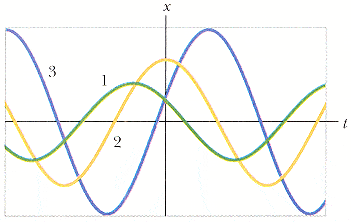# Simple Harmonic motion of the following oscillations (1 Viewer)

### Users Who Are Viewing This Thread (Users: 0, Guests: 1)

#### queenspublic

1. The problem statement, all variables and given/known data

The figure below shows the x(t) curves for three experiments involving a particular spring-box system oscillating in SHM.(a) Rank the curves according to the system's angular frequency, greatest first (use only the symbols > or =, for example 1>2=3).

(b) Rank the curves according to the spring's potential energy at t = 0, greatest first.

(c) Rank the curves according to the box's kinetic energy at t = 0, greatest first.

(d) Rank the curves according to the box's speed at t = 0, greatest first.

(e) Rank the curves according to the box's maximum kinetic energy, greatest first.

2. The attempt at a solution

Clueless, do I have to use the formulas or something to find out which ones are greater?

Last edited:

#### Delphi51

Homework Helper
Re: Oscillations

"angular frequency" is a measure of how many crests or troughs there are per unit time, so (a) is asking first for the one with crests closest together on the graph.

For (b), you need a formula for the potential energy of a spring, hopefully involving the stretch x which is given on the graph. Then you should be able to see what characteristic on the graph determines the PE.

For (c) you must consider the motion of a spring going back and forth repeatedly. When does it have maximum KE? When is the KE zero? You'll want to note those places on each graph.

#### queenspublic

Re: Oscillations

So for (a), what do you mean by crests?

So for (b), you use U= 1/2kx^2?

So for (c), how do I find kinetic energy again?

#### Delphi51

Homework Helper
Re: Oscillations

Read http://www.glenbrook.k12.il.us/GBSSCI/PHYS/CLASS/waves/u10l2a.html [Broken] on crests and troughs.

Yes, use U = 1/2kx^2. It says the spring has the most potential energy when its x is greatest.

An oscillating spring is constantly changing its energy from potential to kinetic and back. The total energy stays the same, so KE + PE = constant total energy. When it has zero PE, it has maximum KE.

Last edited by a moderator:

### The Physics Forums Way

We Value Quality
• Topics based on mainstream science
• Proper English grammar and spelling
We Value Civility
• Positive and compassionate attitudes
• Patience while debating
We Value Productivity
• Disciplined to remain on-topic
• Recognition of own weaknesses
• Solo and co-op problem solving